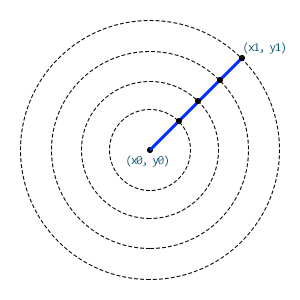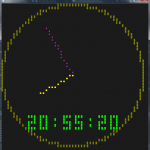## Drawing lines in the Console (or elsewhere)

I remember once needing to code my own drawing functions for an HP48 and not having a clue how to draw line.

My first implementation worked by determining the delta increment that should be applied to both x and y from the starting (x0, y0) point to the final location (x1, y1).
Although this worked, it resulted in horrendously looking jagged lines.
To make matters worst, I also had to calculate the sign of the increments, based on the slope of the line, which added complexity to the code, making it quite ugly and slow.

Then a couple of days later I realized that a line is nothing more than the radius of a circle!
That was quite an eureka moment for me.

Let me explain:To draw line you just need to draw a single point on a circle that increases its size from 0 at the location (x0, y0) up to a radius equal to the line’s length, which can be calculated using Pythagoras’s theorem:

```Dim dx = (x1 - x0)
Dim dy = (y1 - y0)

Dim length As Integer = Math.Sqrt(dx ^ 2 + dy ^ 2)```

And to determine which point to to draw, we just need to get the angle at which the point exists in the circle, again, using Pythagoras:

`Dim angle = Math.Atan2(dy, dx) * Rad2Deg`

Finally, here’s the code that draws that particular point on a circle with a radius that goes from 0 to the line’s length:

```For radius As Integer = 0 To lengthSince that day, this is the way I’ve been coding all my custom line drawing functions.